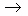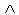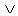Logic
edHelper subscribers - Create a new printable

Number of Keys
 Select the number of different printables: 1 key 2 keys 3 keys 4 keys 5 keys

Number of Pages (for each key)
 Select the number of pages: 1 page 2 pages 3 pages 4 pages

Include an answer key (answer keys will be at the end of the printable)
High School Geometry
High School Geometry
Math

 Name _____________________________Date ___________________
Logic
State the truth value of the following statements.

 1 If p is false and q is false, state the truth for: p(~ pq )
 2 If p is true and q is false, state the truth for:( qp )( q~ p )
 3 If p is false and q is false, state the truth for:(~ qp )( qp )
 4 If p is true and q is false, state the truth for: pq
 5 If p is false and q is true, state the truth for: pq
 6 If p is false and q is true, state the truth for:(~ p~ q )( p~ q )
 7 If p is true and q is true, state the truth for: p( p~ q )
 8 If p is false and q is true, state the truth for:~((~ pq )(~ pq ))
 9 If p is false and q is false, state the truth for: p( qp )
 10 If p is true and q is true, state the truth for:~(( q~ p )( pq ))
 11 If p is false and q is false, state the truth for:(~ pq )( pq )
 12 If p is true and q is false, state the truth for:( p~ q )q
 13 If p is true and q is false, state the truth for:~((~ qp )( p~ q ))
 14 If p is true and q is true, state the truth for: pq
 15 If p is true and q is false, state the truth for: p(~ qp )
 16 If p is false and q is false, state the truth for:~((~ qp )( qp ))
 17 If p is false and q is true, state the truth for:(~ pq )(~ qp )
 18 If p is true and q is false, state the truth for:( qp )q
 19 If p is true and q is true, state the truth for:(~ q~ p )(~ pq )
 20 If p is false and q is true, state the truth for: p(~ qp )

Sample
This is only a sample worksheet.

Logic
edHelper subscribers - Create a new printable

Number of Keys
 Select the number of different printables: 1 key 2 keys 3 keys 4 keys 5 keys

Number of Pages (for each key)
 Select the number of pages: 1 page 2 pages 3 pages 4 pages